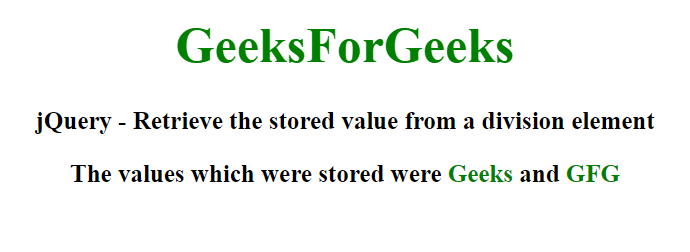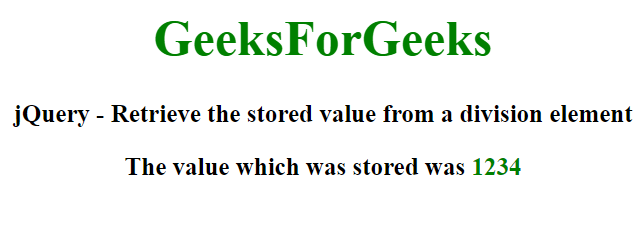Open In App

# How to retrieve stored value from div element using jQuery ?

In this article, we will learn how to retrieve the stored value from any division element using jQuery. This can be used in situations where the value of the division element has to be manipulated or used as per requirements.

Approach 1: We will be using the jQuery data() ,first(), last() and text() methods. We attach or store the data of the division element using the data() method which contains two parameters. The first parameter is the name of the data of the division element and the second parameter is an object literal which consists of two key-value pairs. The value of the first key is “Geeks” and the value of the last key is “GFG“.

Now there are two span elements defined inside the division element which will store the retrieved value. We select the first span element using the first() method and we select the last span element using the last() method. We set their values using the text() method which consists of a single parameter. For the first span element, the value is set to the value of the first key of the object literally defined inside the division element’s data method. Similarly, for the second span element, the value is set to the value of the second key of the object literal.

Example: In this example, we will use the above-explained approach.

## HTML

 ```<``html``>``<``head``>``    ``<``script` `src``=``"https://ajax.googleapis.com/ajax/libs/jquery/3.5.1/jquery.min.js"``>``    ``` `    ````    ``<``style``>``        ``body {``            ``text-align: center;``        ``}` `        ``h1 {``            ``color: green;``            ``font-size: 40px;``        ``}` `        ``div,``        ``p {``            ``font-size: 20px;``            ``font-weight: bold;``        ``}` `        ``span {``            ``color: green;``        ``}``    ````` `<``body``>``    ``<``h1``>GeeksForGeeks` `    ``<``p``>``        ``jQuery - Retrieve the stored value``        ``from a division element``    ``` `    ````    ``<``div``>The values which were stored were``        ``<``span``> and <``span``>``    ``` `    ``<``script` `type``=``"text/javascript"``>` `        ``// Set the value to be stored``        ``\$("div").data("GeeksForGeeks", {``            ``firstKey: "Geeks",``            ``lastKey: "GFG"``        ``});` `        ``// Get the stored value``        ``\$("span").first().text(\$("div")``            ``.data("GeeksForGeeks").firstKey);` `        ``\$("span").last().text(\$("div")``            ``.data("GeeksForGeeks").lastKey);``    ```````

Output:Approach 2: We will be using the jQuery data() and html() methods. This approach is quite similar to the previous approach but the difference is that the html() method is used instead of the text() method. Both these methods return or set the content of the selected element but the html() method is nearly 2 times faster than the text() method. Also instead of two-span elements, there is only one span element that has the stored value. Thus, the second parameter of the data() method is not an object literal here but rather a simple number.

Example: In this example, we are using the above-explained approach.

## HTML

 ```<``html``>``<``head``>``    ``<``script` `src``=``"https://ajax.googleapis.com/ajax/libs/jquery/3.5.1/jquery.min.js"``>``    ``` `    ````    ``<``style``>``        ``body {``            ``text-align: center;``        ``}` `        ``h1 {``            ``color: green;``            ``font-size: 40px;``        ``}` `        ``div,``        ``p {``            ``font-size: 20px;``            ``font-weight: bold;``        ``}` `        ``span {``            ``color: green;``        ``}``    ````` `<``body``>``    ``<``h1``>GeeksForGeeks` `    ``<``p``>``        ``jQuery - Retrieve the stored value``        ``from a division element``    ``` `    ````    ``<``div``>The value which was stored was <``span``>` `    ``<``script` `type``=``"text/javascript"``>``    ` `        ``// Set the value to be stored``        ``\$("div").data("gfgValue", 1234);``        ` `        ``// Get the stored value``        ``\$("span").html(\$("div").data("gfgValue"));``    ```````

Output: